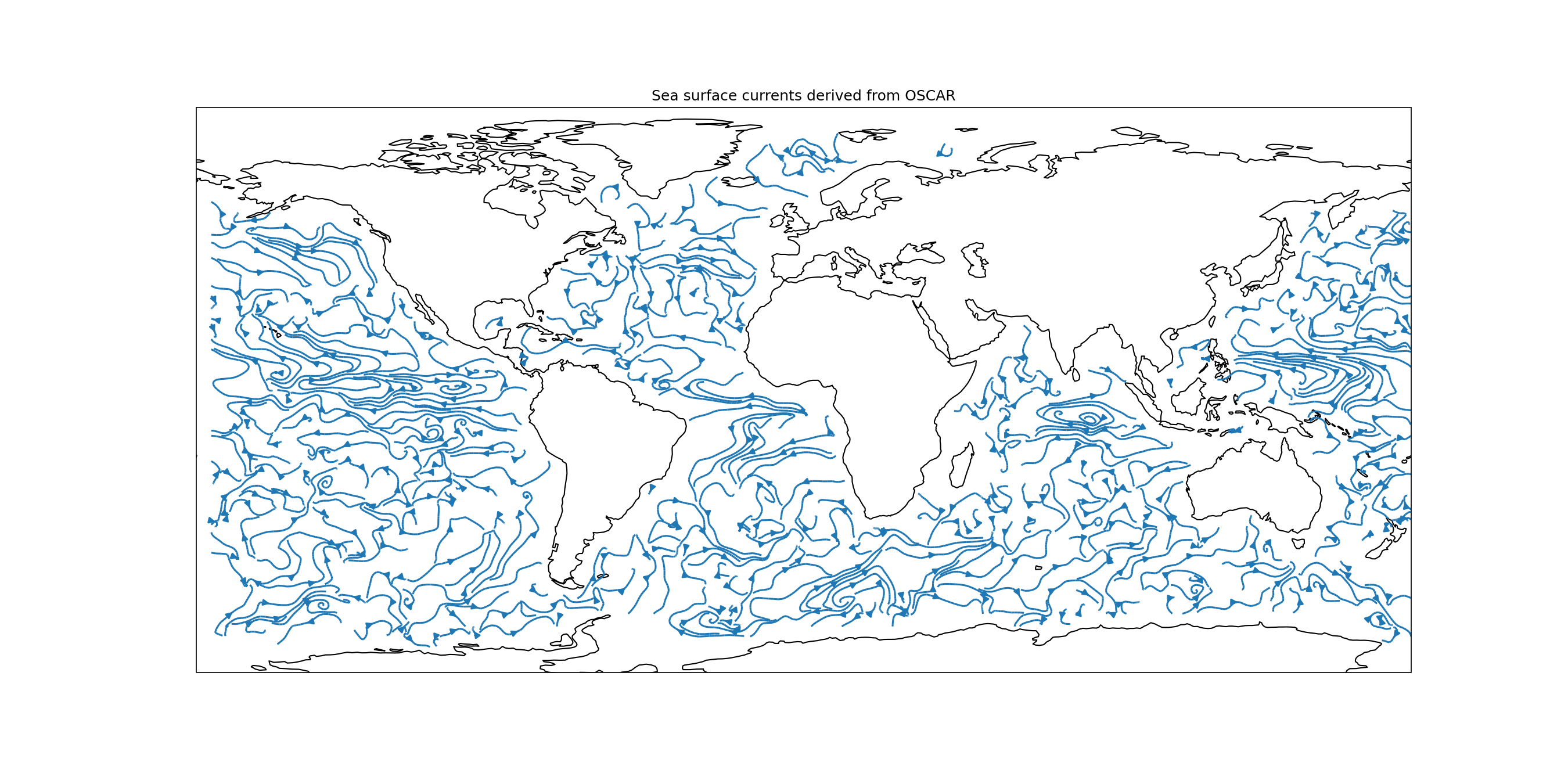“How to plot Ocean Currents with Cartopy”. Reply by Jody Klymak. Oct 20, 2019

Ocean Surface Current Analyses Real-time (OSCAR)

NASA Earth Science Data Systems (ESDS)

import xarray as xr
import numpy as np
import cartopy.crs as ccrs
import matplotlib.pyplot as plt

with xr.open_dataset('oscar_vel2020.nc.gz') as ds:
print(ds)

plt.figure(figsize=(18, 9))

ax = plt.axes(projection=ccrs.PlateCarree()) # plate carrée projection

dec = 10

lon = ds.longitude.values[::dec]

lon[lon > 180] = lon[lon > 180] - 360

mymap = plt.streamplot(
lon,
ds.latitude.values[::dec],
ds.u.values[0, 0, ::dec, ::dec],
ds.v.values[0, 0, ::dec, ::dec],
8,
transform = ccrs.PlateCarree()
)

ax.coastlines()

plt.title('Sea surface currents derived from OSCAR')

plt.savefig("currents18x9.png", dpi=150)

plt.show()